Fit and Confidence Plots

You can use the SGPLOT and SGPANEL procedures to produce fit plots and ellipses (the ellipses plot is available with the SGPLOT procedure only). Fit plots represent the line of best fit (trend line) with confidence limits.
The plot statements include many options for controlling how the output is displayed. The options that are available depend on the plot type. However, some general options apply to most of the fit and confidence plots. For example, options enable you to do the following:
• add confidence limits (CLM) to the plot. When you add CLM limits, you can specify the confidence level, the transparency for the confidence limits, and other visual attributes. You can add CLM limits to loess, penalized B-spline, and regression plots.
• add prediction limits (CLI) for the individual predicted values. When you add CLI limits, you can specify the text that appears for the limits and other visual attributes. You can add CLI limits to penalized B-spline and regression plots.
• control the appearance of the markers and the fit line. You can also specify a smoothing parameter.
• add and customize curve and data labels.
• specify legend labels. You can also show or hide the legend entries for the CLM limits, the CLI limits, and the fit line.
• group the data by the values of a variable. A separate plot is created for each unique value of the grouping variable. The plot elements for each group value are automatically distinguished by different visual attributes.
• specify the value of an ID variable in an attribute map data set. You specify this option only if you are using an attribute map to control visual attributes of the graph.
Note: Not all of these features are available for all of the plots. Also, the list does not include all available options.
The fit and confidence plots are described in the following sections. If you run the examples, your output might differ somewhat depending on the size of your graphics. The examples here were specified to be a particular size using the following statement:
ods graphics on / width=4in;

Ellipse plots create a confidence elliptical curve computed from input data. In order to produce useful output, the ELLIPSE statement should be used with another plot statement that uses numeric axes. Ellipses are available only for the SGPLOT procedure. The SGPANEL procedure does not support ellipses.
The following example shows the relationship of height to weight for a class of students. The example consists of a scatter plot and two ellipses.
Here are the noteworthy features of the example:
• Both ELLIPSE statements use TYPE=PREDICTED. This is the default.
• One ELLIPSE statement uses ALPHA=.2 and the other uses ALPHA=.05.
• The automatically generated legend, which contains an entry for each ellipse and for the scatter plot, has been suppressed. Only the ellipses require a legend.
• A legend was created that contains entries only for the ellipses. Each ELLIPSE statement specifies a legend label and a plot name. The KEYLEGEND statement uses the NAME value to determine the entries in the legend.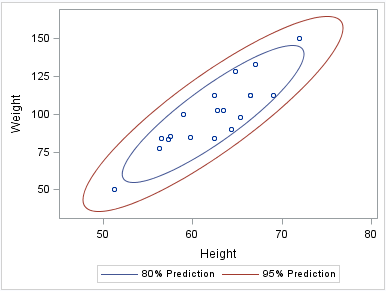proc sgplot data=sashelp.class noautolegend; scatter x=height y=weight; ellipse x=height y=weight / alpha=.2 name="eighty" legendlabel="80% Prediction"; ellipse x=height y=weight / alpha=.05 name="ninetyfive" legendlabel="95% Prediction"; keylegend "eighty" "ninetyfive"; run;

ELLIPSE Statement (SGPLOT procedure)

A loess plot includes a scatter plot of two numeric variables along with an overlaid nonlinear fit line that enables you to perform locally weighted polynomial regression. You can specify the degree of the local polynomials to use for each local regression. You can also change the default smoothing technique that is applied to the fit.
The following examples show the relationship of height to weight and the line of best fit for a class of students. Examples are provided for the SGPLOT and the SGPANEL procedures. In both examples, the automatically generated legend for the fit line is not needed and has been suppressed.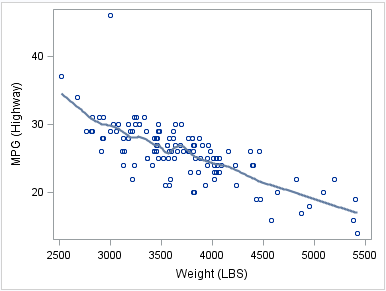proc sgplot data=sashelp.cars noautolegend; where (origin = "Europe"); loess x=weight y=mpg_highway; run;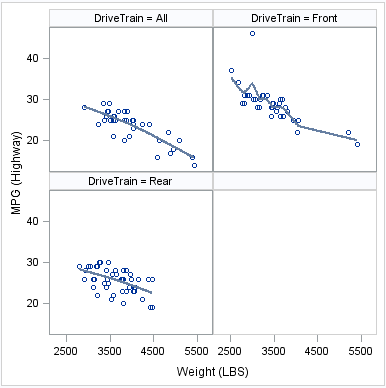proc sgpanel data=sashelp.cars noautolegend; where (origin = "Europe"); panelby drivetrain; loess x=weight y=mpg_highway; run;

LOESS Statement (SGPANEL procedure)
LOESS Statement (SGPLOT procedure)

A penalized B-spline curve includes a scatter plot of two numeric variables along with an overlaid nonlinear fit line. You can specify the degree of the local polynomials to use for each local regression. You can also change the default smoothing technique that is applied to the fit.
The following examples show the relationship of height to weight and the line of best fit for a class of students. Examples are provided for the SGPLOT and the SGPANEL procedures. In both examples, the automatically generated legend for the fit line is not needed and has been suppressed.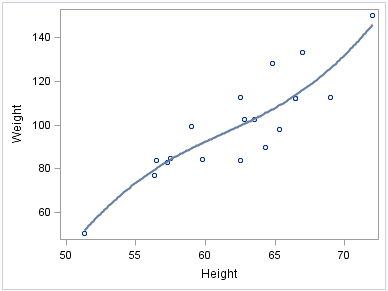proc sgplot data=sashelp.class noautolegend; pbspline x=height y=weight; run;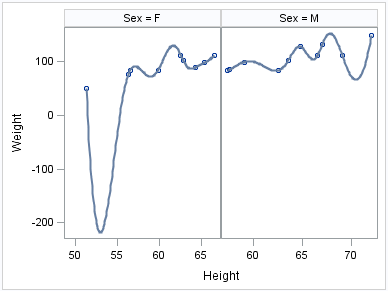proc sgpanel data=sashelp.class noautolegend; panelby sex / uniscale=row; pbspline x=height y=weight; run;

PBSPLINE Statement (SGPANEL procedure)
PBSPLINE Statement (SGPLOT procedure)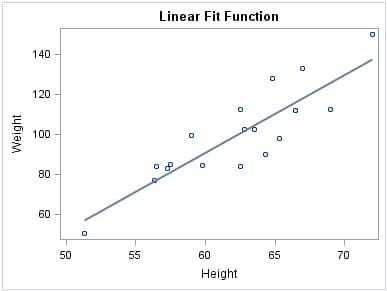title "Linear Fit Function"; proc sgplot data=sashelp.class noautolegend; reg x=height y=weight; run; title;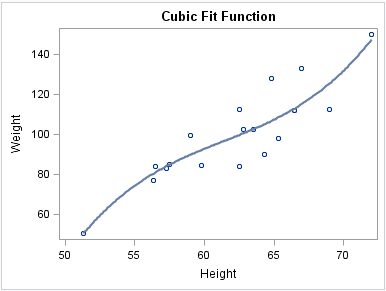title "Cubic Fit Function"; proc sgplot data=sashelp.class noautolegend; reg x=height y=weight / degree=3; run; title;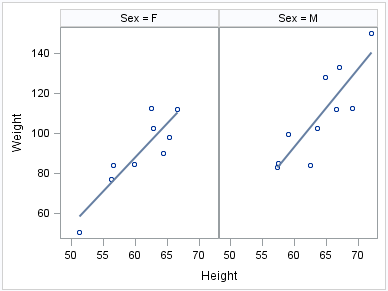proc sgpanel data=sashelp.class noautolegend; panelby sex; reg x=height y=weight; run;